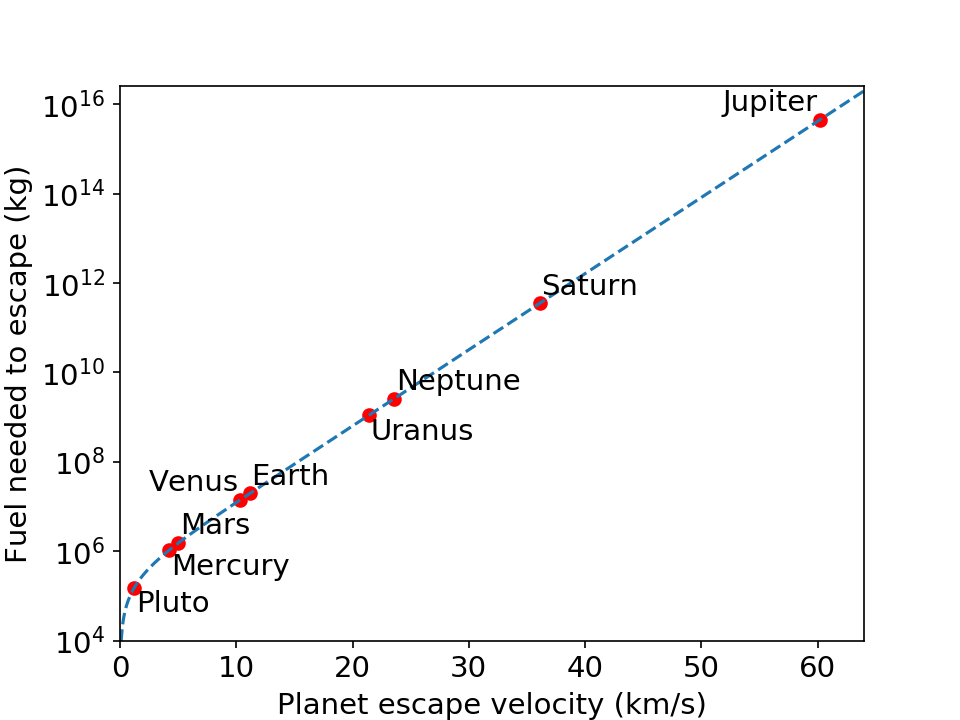Welcome to Project Lovelace! We're still in early development so there are still tons of bugs to find and improvements to make. If you have any suggestions, complaints, or comments please let us know on Discourse, Discord, or GitHub!

# Rocket science

## You will learn about: submitting code, rocket equation.

Moving stuff to outer space is super expensive and takes a lot of energy, which is part of the reason why colonizing the moon or terraforming Mars is extremely hard. To move something heavy into space you need a rocket with enough fuel. But adding fuel makes the rocket even heavier... And if you wanted to visit Mars and come back, you would need enough fuel to leave both Earth and Mars.

We can actually calculate how much fuel a rocket needs using the rocket equation: $m_\mathrm{fuel} = M \left( e^{v/v_e} - 1\right)$ where $M$ is the mass of the rocket (with no fuel), $v_e$ is the exhaust velocity of the rocket, and $e = 2.71828\dots$ is Euler's number. $v$ is the velocity the rocket needs to escape, which is different for every planet. Try to submit some code with a function rocket_fuel(v) that returns $m_\mathrm{fuel}$ for Saturn V ($M = 250,000 \; \mathrm{kg}$, $v_e = 2,550 \; \mathrm{m/s}$) as a function of $v$.Fuel needed for the Saturn V to escape from each planet in our solar system (and yes, Pluto is a dwarf planet). The scale in the y-axis is logarithmic to show the huge differences in fuel needed to escape the different planets. A rocket would need over 100 times more fuel to escape Earth than Pluto. And if you could land on Jupiter, a rocket would need 225 million times more fuel to escape Jupiter than Earth! (Code used to generate this plot can be downloaded from notes after you solve the problem.)

Input: The velocity $v$ the rocket needs to reach to escape the planet in meters per second (m/s).

Output: The mass of fuel $m_\mathrm{fuel}$ needed by the rocket to escape the planet in kilograms (kg).

Example 1

Input: 11186.0 Output: 19843016.2

Example 2

Input: 500.0 Output: 54155.58
 Difficulty rocket_fuel(escape_velocity)

You must be logged in to view your submissions.

### Videos

Why Earth Is A Prison and How To Escape It
Kurzgesagt has a great YouTube video essentially about the rocket equation and why it's so hard to leave Earth

### Notes

• We basically rearranged the Tsiolkovsky rocket equation in this problem. Wikipedia shows a pretty common derivation of the rocket equation.
• At a launch cost of US$1.16 billion (2016 value) and a low Earth orbit payload of 140,000 kg, it cost$8,286 per kg to send stuff to space using the Saturn V.
• The input example uses the escape velocity of Earth (11.186 km/s) and Wikipedia has a list of escape velocities for many other celestial bodies.
• Awesome xkcd graphic showing the depths of gravity wells in our solar system.

### References

F-1 Engine Fact Sheet , NASA Saturn V News Reference (1968)
This is where we sourced the numbers for the Saturn V in this problem. The full Saturn V Press Kit is also online.

Let us know what you think about this problem! Was it too hard? Difficult to understand? Also feel free to discuss the problem, ask questions, and post cool stuff on Discourse. You should be able see a discussion thread below. Would be nice if you don't post solutions in there but if you do then please organize and document your code well so others can learn from it.